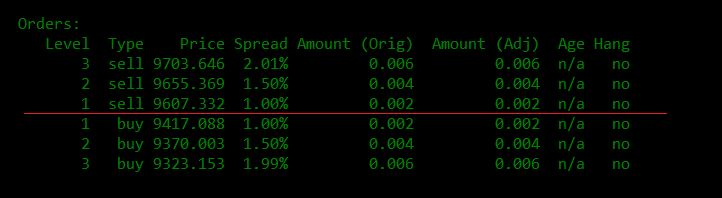# Order Levels¶

These parameters allow you to set multiple levels of orders on each side and gives you more fine-grained control over the spreads and sizes of each set of orders.

## order_levels¶

The number of order levels to place for each side of the order book.

Prompt:

How many orders do you want to place on both sides?
>>>


## order_level_amount¶

The size can either increase (if set to a value greater than zero) or decrease (if set to a value less than zero) for subsequent order levels after the first level.

Prompt:

How much do you want to increase or decrease the order size for each additional order?
>>>


## order_level_spread¶

The incremental spread increases for subsequent order levels after the first level.

Prompt:

Enter the price increments (as percentage) for subsequent orders?
>>>


Warning

Setting order_level_spread to a very low number may cause multiple orders to be placed on the same price level. For example, for an asset like SNM/BTC, if you set an order interval percent of 0.4 (~0.4%) because of low asset value, the price of the next order will be rounded to the nearest price supported by the exchange, which in this case might lead to multiple orders being placed at the same price level.

## How it works¶

Enter the value for order_levels to specify how many orders you want to place on each side (buy and sell).

Note

Example: Entering 3 places three bid and three ask orders on each side of the book, for a total of 6 orders.

Users can also increase or decrease the size of subsequent orders starting from the first order in increments or decrements specified in order_level_amount. This can be set to 0 if you don't want your multiple order sizes to increase Greater than 0(i.e., 0.4) to allow order sizes to increase by 0.4 after the first level Less than 0(i.e., -2) to enable order sizes to decrease by 2 after the first level.

Note

Example: If the order start size is 7000 and the order step size is 1000, the second-order size is 8000, and the third-order is 9000.

The order_level_spread logic works the same as the order step size, but instead, it increases the spreads of your subsequent orders starting from the first order.

Note

Example: The spread of your first buy and sell order is 1 (1%), and your order interval amount is 2 (2%). The spread of your second order is 3 (3%), and the third-order is 5 (5%).

Let us focus on one side of the order for now: the "sell" side of the order book. Given the sample scenarios above, your active orders will show as:## Sample configuration¶

- market: BTC-USDT
- order_amount: 0.002
- order_levels: 3
- order_level_amount: 0.002

Running a bot with the parameters above, the status command shows 3 levels of orders in the BTC-USDT trading pair: